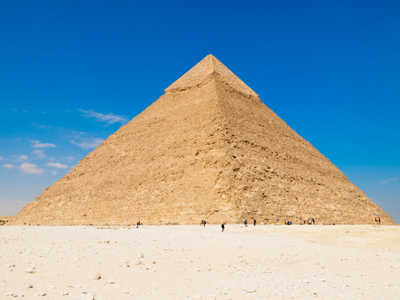A pyramid has a square face and four triangular faces.

# Shapes (Year 3)

In KS2 Maths, you'll explore cool shapes! Year three is all about 2D and 3D shapes like circles, triangles, and cubes. Get ready for pentagons, hexagons, spheres, cylinders, and pyramids! Learn fancy words like vertices, edges, and faces.

Shapes have angles, faces, and edges. Some are flat (2D) like triangles, and some are solid (3D) like cubes. Exciting, right? Do you have a favourite shape?

How much do you know about shapes? Can you spot the difference between squares and cubes? What are vertices? Test your shape skills in this fun quiz!

1.
How many faces does a cube have?
2
4
6
8
Every face is a square
2.
Which shape is half of a circle?
Semicircle
Hemisphere
Oval
Prism
A hemisphere is half of a sphere
3.
Which shape has only one edge?
Square
Circle
Triangle
Pentagon
A circle has no vertices
4.
Which shape has a square face and four triangular faces?
Prism
Cylinder
Pyramid
Sphere
The pyramids in Egypt are ancient buildings which are the shape of a pyramid
5.
What is the name of the shape that resembles a ball?
Hemisphere
Cone
Sphere
Semicircle
A sphere is like a 3 dimensional circle
6.
Which 3D shape has three faces and two edges?
Cube
Cylinder
Prism
Cone
A cylinder is like a tube with circles at each end
7.
What is the name for all polygons with 4 sides?
Square
Cube
Rectangle
A square and a rectangle are both quadrilaterals
8.
How many sides does a hexagon have?
5
6
7
8
One way to remember this is that both heXagon and siX have a letter X in them
9.
What are the corners of a shape also known as?
Faces
Angles
Edges
Vertices
The singular term for vertices is vertex
10.
What is the name of the shape with five sides?
Pentagon
Hexagon
Octagon
Cylinder
Pentagons have 5 sides and a pentagram is a 5 pointed star